# Creating & Interpreting Dot Plots: Process & Examples

An error occurred trying to load this video.

Try refreshing the page, or contact customer support.

Coming up next: Creating & Interpreting Box Plots: Process & Examples

### You're on a roll. Keep up the good work!

Replay
Your next lesson will play in 10 seconds
• 0:01 Using Dot Plots
• 0:32 What Is a Dot Plot?
• 1:40 Creating a Dot Plot
• 3:26 Interpreting a Dot Plot
• 5:47 Lesson Summary

Want to watch this again later?

Timeline
Autoplay
Autoplay
Speed

#### Recommended Lessons and Courses for You

Lesson Transcript
Instructor: Cathryn Jackson

Cat has taught a variety of subjects, including communications, mathematics, and technology. Cat has a master's degree in education and is currently working on her Ph.D.

Dot plots are a visual way to display the frequency distribution in a data set. In this lesson, you will learn how to construct a dot plot and understand its uses.

## Using Dot Plots

Jason is a writer for a local magazine. He is collecting research about some of the trends among college students. Jason asks the students about their favorite study snacks, what they do to relax, how many hours they spend studying and how many hours they work during the week. Jason wants to organize this information in a visual way that shows the popularity of each choice. Jason can do this by creating a dot plot.

In this lesson, learn how to create and interpret a dot plot to visually analyze your data.

## What Is a Dot Plot?

A dot plot is a visual representation of data using intervals or categories of variables; the dots represent an observation in the data.

A dot plot can be used to analyze frequency in a data distribution. Jason's data is all about frequency. He is asking questions about the frequency, or amount, of students that select each option. A dot plot can quickly and easily tell you how popular a certain choice or item is in your data set. Take a look at the results of Jason's survey:Notice that there are two distinct types of data. There are numbers, such as the number of study hours or the number of work hours; and there are things or categories, such as the snacks and the relaxation activities.

These two different types of data are categorical and numerical. Categorical data is data that is grouped by type or object, not by numbers. Numerical data is information that is measurable. It is always collected in number form. Let's look at different examples of numerical and categorical data when creating a dot plot.

## Creating a Dot Plot

When creating a dot plot, first label all of the data categories. If you are working with categorical data, then this will be the options that were given in the data set. For example, Jason asked the college students which snacks they prefer. Therefore, the categories in this dot plot would be Twizzlers, Payday, Doritos, Honey Buns, Whoppers, Hershey and Skittles.

Next, place a divider line over the top of your categories. This will separate the data from the labels.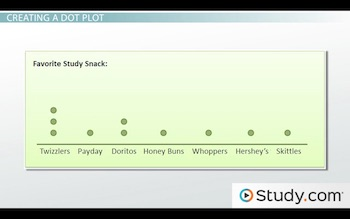Finally, place a dot for each selection in your data set. You'll notice that there are three dots above the Twizzlers option. Therefore, three people selected Twizzlers as their favorite study snack. Each dot represents one person that selected that option.

This is Jason's dot plot for what the college students do to relax: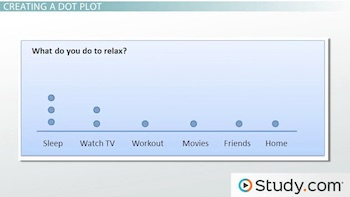Notice that three students selected sleep as their preferred method of relaxation, two students selected watch TV and one student each selected go to the movies, hang out with friends and go home.

Notice that this dot plot contains number labels rather than categories: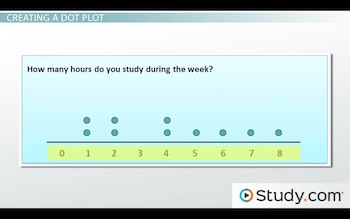That is because this data set is numerical data rather than categorical. Each number represents the number of hours each student spends studying each week. Each dot represents the student that chose that option. Notice that there are no dots on the zero and three labels. That means that no students selected zero or three hours as the number of hours they spend each week studying.

This is another example of numerical data: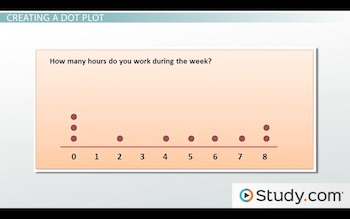This dot plot represents the data set when Jason asked the students how many hours they worked each week. Notice that three students selected zero, which would likely tell Jason that they do not work.

## Interpreting a Dot Plot

Jason is now conducting a second interview for a follow up article. His readers want to know the following information:

1. Ages of 20 college students surveyed
2. Number of class hours each student was taking
3. Amount of money spent on books in this semester

When you are analyzing dot plots with numerical data, you can analyze the shape of that particular dot plot. Your dot plot may be symmetrical or skewed.

Symmetry is where the shape created is mirrored nearly perfectly across a line. In statistics, you'll find that visual representations of data will be nearly perfect, but not always perfect. That is why we define symmetry as being nearly perfect. When a data set is symmetrical, then the mean, median and mode all occur in the same point. The mean is the average. The median is the middle value. The mode is the number that appears most.

This is an example of a dot plot that is symmetrical: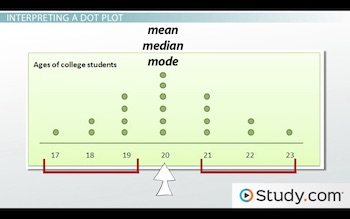Notice that the middle option is the tallest, and each side of the middle option mirrors the other side. This tells us that the mean, median and mode of Jason's data set are 20.

To unlock this lesson you must be a Study.com Member.

### Register to view this lesson

Are you a student or a teacher?

### Unlock Your Education

#### See for yourself why 30 million people use Study.com

##### Become a Study.com member and start learning now.
Back
What teachers are saying about Study.com

### Earning College Credit

Did you know… We have over 160 college courses that prepare you to earn credit by exam that is accepted by over 1,500 colleges and universities. You can test out of the first two years of college and save thousands off your degree. Anyone can earn credit-by-exam regardless of age or education level.